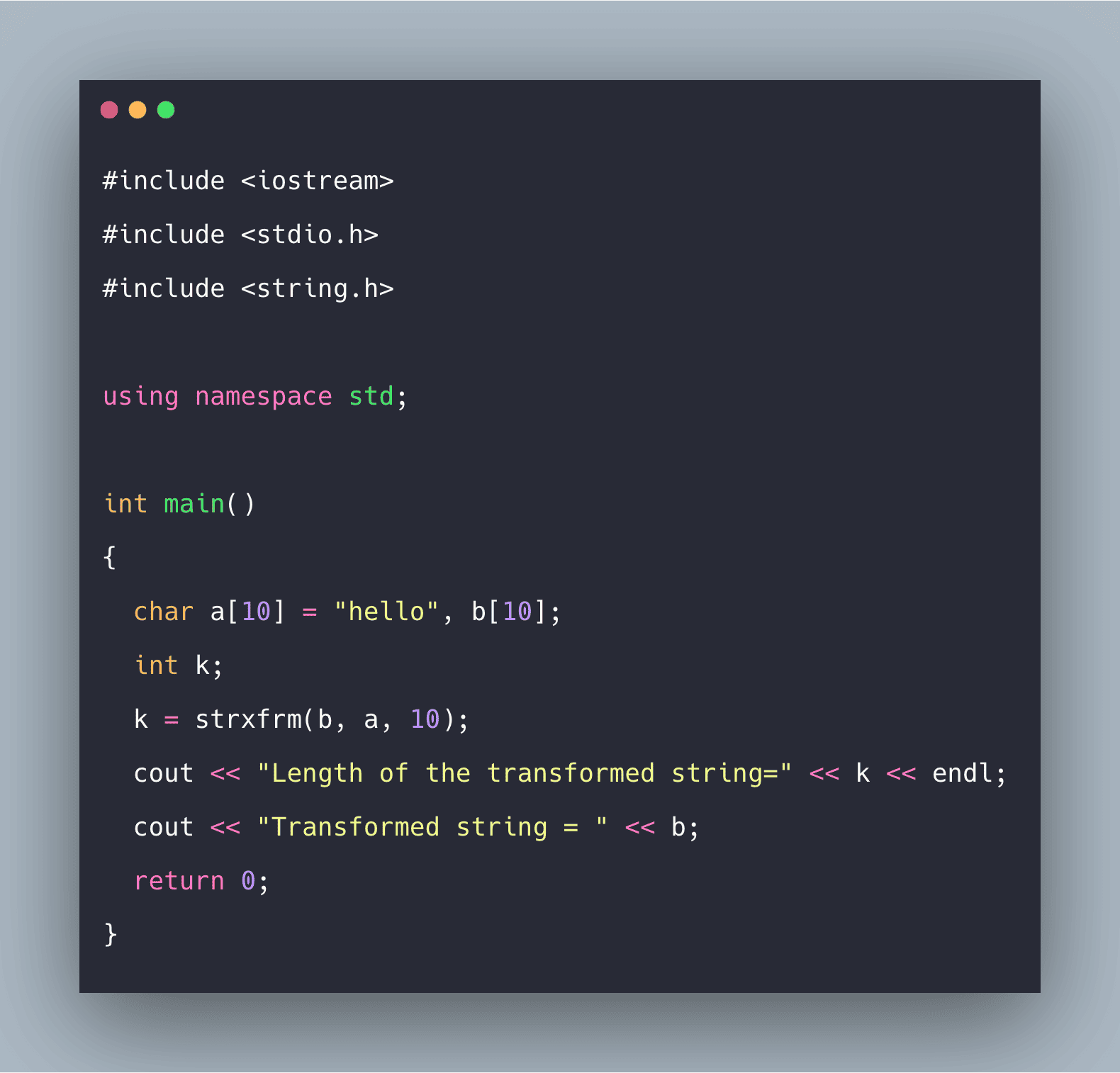# C++ strxfrm() Function Example | strxfrm() in C++C++ strxfrm() library converts a given string into an implementation-defined form. It converts the string into a current locale, and then it is placed in the defined form.

C++ strxfrm() library converts a given string into an implementation-defined form. It converts the string into a current locale, and then it is placed in the defined form. The transformation is done in such a way that after transformation of two strings, the strings shown by  strcmp() and  strcoll() on the given two strings are the same.

### C++ strxfrm()

The C/C++ library strxfrm() transform the characters of source string into the current locale and place them in the destination string. For that LC_COLLATE category is used, which is defined in** locale.h**. The strxfrm() function performs transformation in such a way that the result of strcmp on two strings is the same as the result of strcoll on two original strings.

For Example, suppose we take two strings str1 and str2, and after transforming string using strxfrm(), it becomes strA and strB. Then if we call strcmp() by passing strA and strB as parameters, respectively, we will get the same results as by calling strcoll() by passing str1 and str2 as parameters.

#### Syntax

``size_t strxfrm(char *a, const char *b, size_t num);``

In the above syntax, the function converts the total ‘num’ characters of the given string pointed to by ‘b’ to a defined form, and finally, it is stored in the location, which is pointed by a.

## Dicey Issues in C/C++

C/C++ problems. If you are familiar with C/C++then you must have come across some unusual things and if you haven’t, then you are about to. The below codes are checked twice before adding, so feel free to share this article with your friends.

## Loops in C++ | For, While, and Do While Loops in C++

In this Video We are going to see how to use Loops in C++. We will see How to use For, While, and Do While Loops in C++.

## Using isdigit() in C/C++

In this article, we'll take a look at using the isdigit() function in C/C++. This is a very simple way to check if any value is a digit or not. Let's look

## Object Oriented Programming in C++ | C++ OOPs Concepts | Learn Object Oriented C++

C++ is general purpose, compiled, object-oriented programming language and its concepts served as the basis for several other languages such as Java, Python, Ruby, Perl etc.